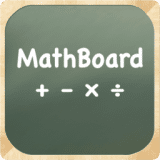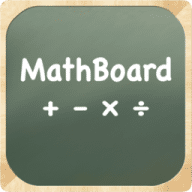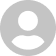MathBoard
3.4.0

0.0# MathBoard

3.4.0
20 October 2019

Learn math the fun and easy way.

## Overview

MathBoard is a fantastic math learning tool for OS X. MathBoard is appropriate for all ages from kindergarten (with simple addition and subtraction problems) to elementary school where learning multiplication and division can be a challenge. You can control the range of numbers you want to work with, the number of questions you want to answer, and even assign a time limit per quiz. MathBoard will make learning math fun.

##### Features
• Random problem generation (up to 250 questions per quiz)
• Addition, subtraction, multiplication, division, squares, cubes and square roots
• Number ranges are configurable from -1000 to 1000, including the ability to require certain numbers to be in each problem
• Intelligent "wrong" answer generation makes guessing more difficult; includes the ability to omit negative answers
• A quiz can be timed, and timing can be set as a countdown timer or elapsed time
• A quiz can be saved, making it simple to replay a similar quiz, or the same quiz, at a later date
• Wrong answers are reviewable with both the incorrect and correct answer visible (and equation displayed); you can even generate a quiz from just the wrong answers
• Problem Solver for addition, subtraction, multiplication and division problems
• Quick reference math tables for addition, subtraction and multiplication

## What's new in MathBoard

Version 3.4.0:
• Updated for macOS 10.15 (Catalina)

#### Join over 500,000 subscribers.

Subscribe for our newsletter with best Mac offers from MacUpdate.

## 1 MathBoard Reviews

Rate this app:24 June 2011

"Kindergarten...to elementary school" ≠ "Math learning tool for all ages"
Like
Version 1.1.424 June 2011
"Kindergarten...to elementary school" ≠ "Math learning tool for all ages"
Like
Version 1.1.4